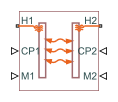# Specific Dissipation Heat Transfer

Simple heat transfer model between two general fluids

•Libraries:
Simscape / Fluids / Fluid Network Interfaces / Heat Exchangers / Fundamental Components

## Description

The Specific Dissipation Heat Transfer block models the heat transfer between two fluids given only minimal knowledge of component parameters. The fluids are controlled by physical signals, with these providing the entrance mass flow rate and isobaric specific heat for each. Thermal ports set the entrance temperatures of the fluids.

The rate of heat transfer is calculated from the specific dissipation, a parameter specified in tabulated form as a function of the entrance mass flow rates. The specific dissipation quantifies the amount of heat exchanged between the fluids per unit of time when the entrance temperatures differ by one degree.

Pressure losses and other aspects of flow mechanics are ignored. To capture such effects, use the heat exchanger interface blocks provided in the same library. Combine heat transfer and heat exchanger blocks to model a custom heat exchanger. See the composite block diagrams of the heat exchanger blocks for examples.

### Heat Transfer Rate

Heat flows from the warmer fluid to the cooler fluid, at a rate proportional to the difference between the fluid entrance temperatures. The heat flow rate is positive if fluid 1 enters at a higher temperature than fluid 2—and therefore if heat flows from fluid 1 to fluid 2:

`$Q=SD\left({T}_{1,\text{in}}-{T}_{2,\text{in}}\right),$`

where T*,in are the fluid entrance temperatures, determined by the conditions at thermal port H1 for fluid 1 and H2 for fluid 2. SD is the specific dissipation obtained from the specified tabulated data at the given mass flow rates:

`$SD=SD\left({\stackrel{˙}{m}}_{1},{\stackrel{˙}{m}}_{2}\right),$`

where $\stackrel{˙}{m}$ are the entrance mass flow rates, specified through physical signal port M1 for fluid 1 and M2 for fluid 2. The specific dissipation can be calculated for a set of entrance mass flow rates (${\stackrel{˙}{m}}_{1}$, ${\stackrel{˙}{m}}_{2}$) given the experimental values of the heat transfer rate and the corresponding entrance temperature difference:

`$SD=\frac{Q}{{T}_{1,in}-{T}_{2,in}}.$`

Maximum Heat Transfer Rate

The heat transfer rate is constrained so that the specific dissipation used in the calculations can never exceed the maximum value:

`$S{D}_{\text{max}}=\text{min}\left({C}_{1},{C}_{2}\right),$`

where C* are the thermal capacity rates of the controlled fluids, each defined as:

`${C}_{*}={\stackrel{˙}{m}}_{*}{c}_{p,*},$`

with cp,* denoting the isobaric specific heat of the fluid, specified through physical signal port CP1 for fluid 1 and CP2 for fluid 2. The constraint on the maximum heat transfer rate is implemented in the form of a piecewise function:

A warning is issued whenever the heat flow rate exceeds the maximum value, $S{D}_{max}\left({T}_{1,in}-{T}_{2,in}\right)$, if the Check if violating maximum specific dissipation block parameter is set to `Warning`.

## Ports

### Input

expand all

Isobaric specific heat of controlled fluid 1.

Isobaric specific heat of controlled fluid 2.

Entrance mass flow rate of controlled fluid 1. Positive values indicate flow into the heat exchanger. Negative values indicate flow out of the heat exchanger.

Entrance mass flow rate of controlled fluid 2. Positive values indicate flow into the heat exchanger. Negative values indicate flow out of the heat exchanger.

### Conserving

expand all

Entrance temperature of controlled fluid 1.

Entrance temperature of controlled fluid 2.

## Parameters

expand all

Array of mass flow rates at the inlet for controlled fluid 1. Each value corresponds to a row in the specific dissipation lookup table. Positive values indicate flow into the heat exchanger and negative values indicate flow out of the heat exchanger.

Array of mass flow rates at the inlet for controlled fluid 2. Each value corresponds to a row in the specific dissipation lookup table. Positive values indicate flow into the heat exchanger and negative values indicate flow out of the heat exchanger.

Matrix of specific dissipation values corresponding to the specified mass flow rate arrays for controlled fluids 1 and 2. The block uses the tabulated data to calculate the heat transfer at the simulated operating conditions.

Mass flow rate below which to initiate a smooth flow reversal to prevent discontinuities in the simulation data.

Option to warn if the specific dissipation exceeds the maximum value described in the block description.

## Version History

Introduced in R2017b